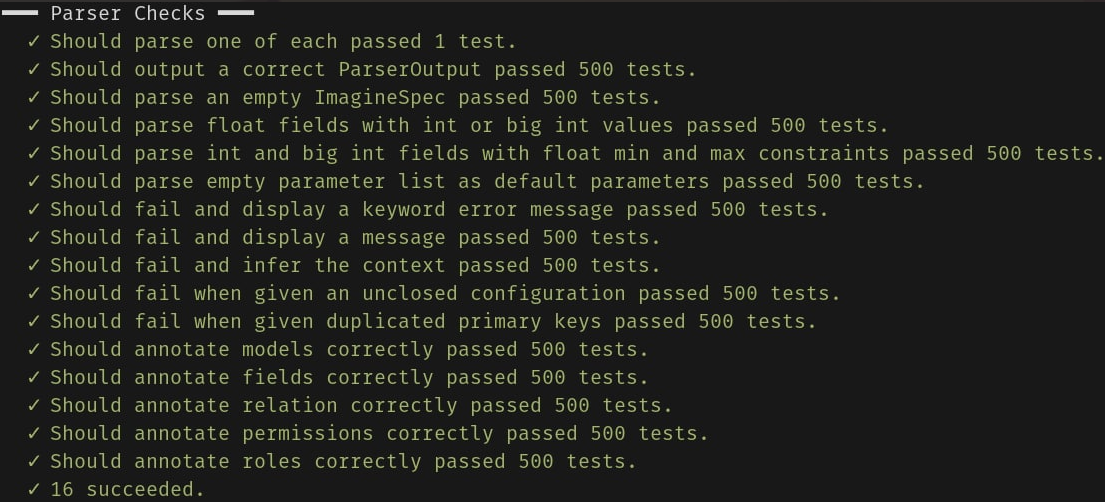# How to test a compiler in Haskell

#### Tomaz Gomes Mascarenhas

Imagine Compilers Team

### The Problem#

As we develop a compiler, like any other piece of software, it is crucial to implement high quality tests. But testing a compiler is not a trivial task - even if the source language is a DSL and not a complex general purpose programming language, creating test cases and checking if the compiler's output is correct can be very challenging. Let's consider the first phase of the compiler: parsing. We can understand this phase as a function from a string, representing some code in the source language, to our internal data structure that stores all relevant information. For instance, let's consider that we are only storing models and relations, and let's consider the following input for our parser:

model Person {
name string [primary key]
}
model Car {
plate string [primary key]
}
relationship Owns {
one owner from Person
many cars from Car
}

The parser will take this specification as input and produce the corresponding data structure. In case of Imagine, we choose to represent this information as an Algebraic Data Type of Haskell, which contains a list of models and a list of relationships:

data DataModelSpec =
DataModelSpec { models :: [Model]
, relations :: [Relationship]
}

So, for the example above, our parser should produce as output something like:

DataModelSpec { models = [Person, Car]
, relations = [One Person <-> Many Cars]
}

We are omitting the details of the datatypes Model and Relationship for brevity. So, to test the parser, we must produce strings that represent valid inputs, like the example above, execute the parser on it and check if the output is correct. But how could we know that the output is correct? The only tool that we have to obtain the DataModelSpec from an input is the parser, and we obviously can't use him to test itself.

### First Approach#

The first and more straightforward way to solve this problem is to use some predefined set of test cases. This way we can manually find the expected output of each input and store them in the tests. Then we just need to check if the output of the parser matches the stored values.

Although this approach works, it has the disadvantage of being static: we will only be able to cover the corner cases that we can think about. There are all kinds of corner cases: what if there is a relation in which the two models are the same? What if there is two models with the same name? What if there is a model that doesn't exist specified in one of the relations? Our parser must behave correctly in every one of these situations. If we miss some of these corner case in the predefined tests, we can by accident accept a wrong implementation of the compiler.

Another issue with this approach is that we can’t test the performance of our compiler in larger cases, unless we put in a big amount of effort to write them by hand.

### Second Approach#

To address this issue, we need to find a way to generate random tests. This way, if we generate a large number of them, there is a good chance that each corner case will appear at least once. But, as we discussed, we can't generate directly the input strings for the parser, since we wouldn't know if the output was correct. What we decided to do instead, was to generate random DataModelSpec's, using the Hedgehog library in Haskell. Also, we created a function that, given a DataModelSpec, produces one input string that corresponds to it (essentially, the inverse function of our parser). This way, we can check our compiler by generating a large number of random DataModelSpec's, applying this new function to each one of them and run the parser over the output of the function. If the output of the parser is equal to the original DataModelSpec, then our parser is working correctly, otherwise it is not.Of course, this approach has the disadvantage that we need to trust that we implemented the function to convert form DataModelSpec to string correctly, but this function is far more simple than our parser.

The following snippet shows one of our tests, that implements this strategy:

parseCorrectly :: Spec
parseCorrectly =
it "Should output a correct ParserOutput" \$ do
(inp, expected) <- H.forAll validInput
case parse "" inp of
Left e -> failsWithParseError expected inp e
Right parsed -> H.diff parsed cmpParserOutput expected

### Conclusion#

Testing a compiler can be challenging due to the complexity of it’s output. In this article we shared a simple technique that can be very helpful to deal with this problem and that we use at Imagine in our tests, which we believe makes our compiler safer.